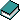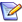# Simultaneous equation modelSimultanes Gleichungssystem   (german)

used for: Recursive model, Simultaneous equations, Simultaneous equations model